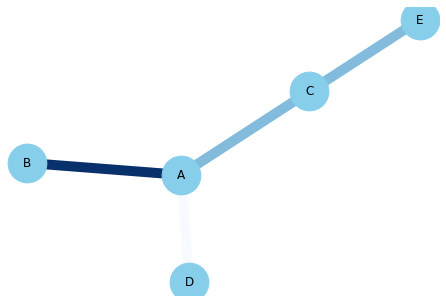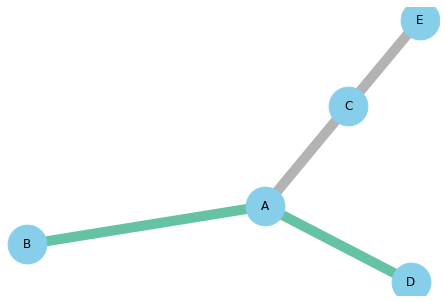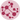# Map Color to the Edges of a Network

This post follows the post #324 where you can see how to map a color to each nodes of a network. You can find out how to map colors to the edges of a network chart in this post.

This time, we suppose that we have a feature for each edge of our network. For example, this feature can be the amount of money that this links represents (numerical value), or on which continent it happened (categorical value). We want the edge to be different according to this variable, and here is how to do it:

## Numerical

``````# libraries
import pandas as pd
import numpy as np
import networkx as nx
import matplotlib.pyplot as plt

# Build a dataframe with your connections
df = pd.DataFrame({ 'from':['A', 'B', 'C','A'], 'to':['D', 'A', 'E','C'], 'value':[1, 10, 5, 5]})

G=nx.from_pandas_edgelist(df, 'from', 'to', create_using=nx.Graph() )

# Custom the nodes:
nx.draw(G, with_labels=True, node_color='skyblue', node_size=1500, edge_color=df['value'], width=10.0, edge_cmap=plt.cm.Blues)``````## Categorical

``````# libraries
import pandas as pd
import numpy as np
import networkx as nx
import matplotlib.pyplot as plt

# Build a dataframe with your connections
df = pd.DataFrame({ 'from':['A', 'B', 'C','A'], 'to':['D', 'A', 'E','C'], 'value':['typeA', 'typeA', 'typeB', 'typeB']})

# And I need to transform my categorical column in a numerical value typeA->1, typeB->2...
df['value']=pd.Categorical(df['value'])
df['value'].cat.codes👋 This document is a work by Yan Holtz. Any feedback is highly encouraged. You can fill an issue on Github, drop me a message onTwitter, or send an email pasting `yan.holtz.data` with `gmail.com`.